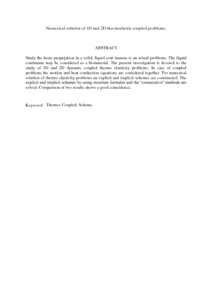# Numerical solution of 1D and 2D thermoelastic coupled problems.

## Citation

Khaldjigitov, Abduvali and Qalandarov, Aziz and Nik Long, Nik Mohd Asri and Eshkuvatov, Zainidin K. (2012) Numerical solution of 1D and 2D thermoelastic coupled problems. International Journal of Modern Physics: Conference Series, 9 (2012). pp. 503-510. ISSN 2010-1945

## Abstract

Study the heats propagation in a solid, liquid cont inuums is an actual problems. The liquid continuum may be considered as a biomaterial. The present investigation is devoted to the study of 1D and 2D dynamic coupled thermo elasticity problems. In case of coupled problems the motion and heat conduction equations are considered together. For numerical solution of thermo elasticity problems an explicit and implicit schemes are constructed. The explicit and implicit schemes by using recurrent formulas and the “consecutive” methods are solved. Comparison of two results shows a good coincidence.Preview
PDF (Abstract)
Numerical solution of 1D and 2D thermoelastic coupled problems.pdfView Item